Estimating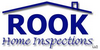The process of calculating the cost of a project. This can be a formal and exact process or a quick and imprecise process.
Found on http://www.encyclo.co.uk/local/20933

Estimating• (p. pr. & vb. n.) of Estimate
Found on http://thinkexist.com/dictionary/meaning/estimating/

Estimating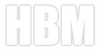- The process of calculating the cost of a project. This can be a formal and exact process or a quick and imprecise process.
Found on http://www.homebuildingmanual.com/Glossary.htm

Estimating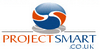Estimating uses a range of tools and techniques to produce estimates. An estimate is an approximation of a projects timescale and cost that is refined throughout the project.
Found on http://www.projectsmart.co.uk/glossary.html

Estimating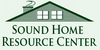The process of calculating the cost of a project. This can be a formal and exact process or a quick and imprecise process.
Found on http://www.soundhome.com/glossary

Estimating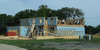The process of calculating the cost of a project. This can be a formal and exact process or a quick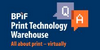To calculate the costs of a job.